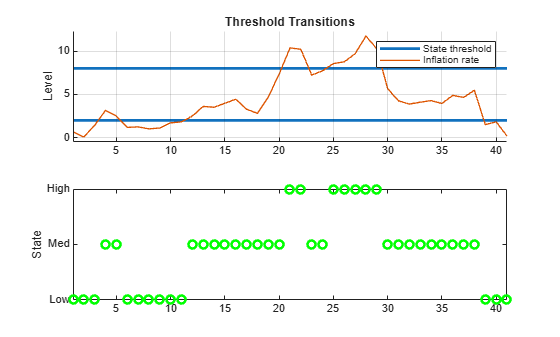# ttstates

Threshold variable data state path

## Syntax

``states = ttstates(tt,z)``

## Description

example

````states = ttstates(tt,z)` returns the state path for values of the threshold variable `z` relative to the levels in the `Levels` property of `tt`.```

## Examples

collapse all

Load the yearly Canadian inflation and interest rates data set. Extract the inflation rate based on consumer price index (`INF_C`) from the table.

```load Data_Canada INF_C = DataTable.INF_C;```

Assume the following characteristics of the inflation rate series:

• Rates below 2% are low.

• Rates at least 2% and below 8% are medium.

• Rates at least 8% are high.

• States transition abruptly.

Create threshold transitions to describe the Canadian inflation rates.

```statenames = ["Low" "Med" "High"]; tt = threshold([2 8],StateNames=statenames);```

Infer the state path by passing the inflation rate series through the threshold transitions.

```n = numel(INF_C); states = ttstates(tt,INF_C); snpath = tt.StateNames(states);```

`states` is an `n`-by-1 vector of inferred state indices. `snpath` is the state path using state names instead of indices.

Separately plot the inflation rate series and inferred state path.

```figure tiledlayout(2,1) nexttile h = ttplot(tt,Data=INF_C); legend(h([1 3]),["State threshold" "Inflation rate"]) nexttile plot(states,'go',LineWidth=2) ylabel('State') yticks(1:3) yticklabels(tt.StateNames) axis tight```## Input Arguments

collapse all

Threshold transitions, with `NumStates` states, specified as a `threshold` object. `tt` must be fully specified (no `NaN` entries).

Threshold variable data, specified as a numeric vector.

Data Types: `double`

## Output Arguments

collapse all

Threshold data states, returned as a numeric vector with the same length as `z`.

If the transition mid-levels `tt.Levels` are t1, t2,… tn, `ttstates` labels states (−∞,t1), [t1,t2),… [tn,∞) as `1`, `2`, …, `n+1`, respectively.

States are independent of threshold rates.

## Algorithms

In threshold-switching dynamic regression models (`tsVAR`), state transitions occur when a threshold variable crosses a transition mid-level. Discrete transitions result in an abrupt change in the submodel computing the response. Smooth transitions create weighted combinations of submodel responses that change continuously with the value of the threshold variable, and state changes indicate a shift in the dominant submodel. For more details, see `tsVAR`.

## Version History

Introduced in R2021b